# Measures of Dispersion solutions inter maths IIA

Intermediate mathematics IIA solutions for chapter 8, Measures of dispersion exercise 8(a)

Read the text book lesson Measures of Dispersion very well.

Then observe the example problems and solutions in the text book. Try them well.

The given solutions are very easy to understand. Observe the solutions and try them in your own method.

You can also see the solutions for

Complex numbers solutions for textbook

De Moivre’s Theorem textbook solutions

Exercise 3(b)

Exercise 3(c)

You can see solutions for IIB

1. Circle

3. Parabola

4. Ellipse

You can see the solutions for first year maths 1A and 1B for examination purpose

Inter maths 1A solutions

3. Matrices

You can also see the solutions for 1b

1. Locus

The straight line sa

Straight lines la

Maths 1b textbook solutions

Locus

Exercise 1(a)

Transformation of axes

Exercise 2(a)

The Straight Line

The Plane

Exercise 7(a)

Limits and Continuity

Exercise 8(a)

Exercise 8(b)

M

# Inter maths solutions for Measures of Dispersion

Measures of Dispersion – Exercise 8(a)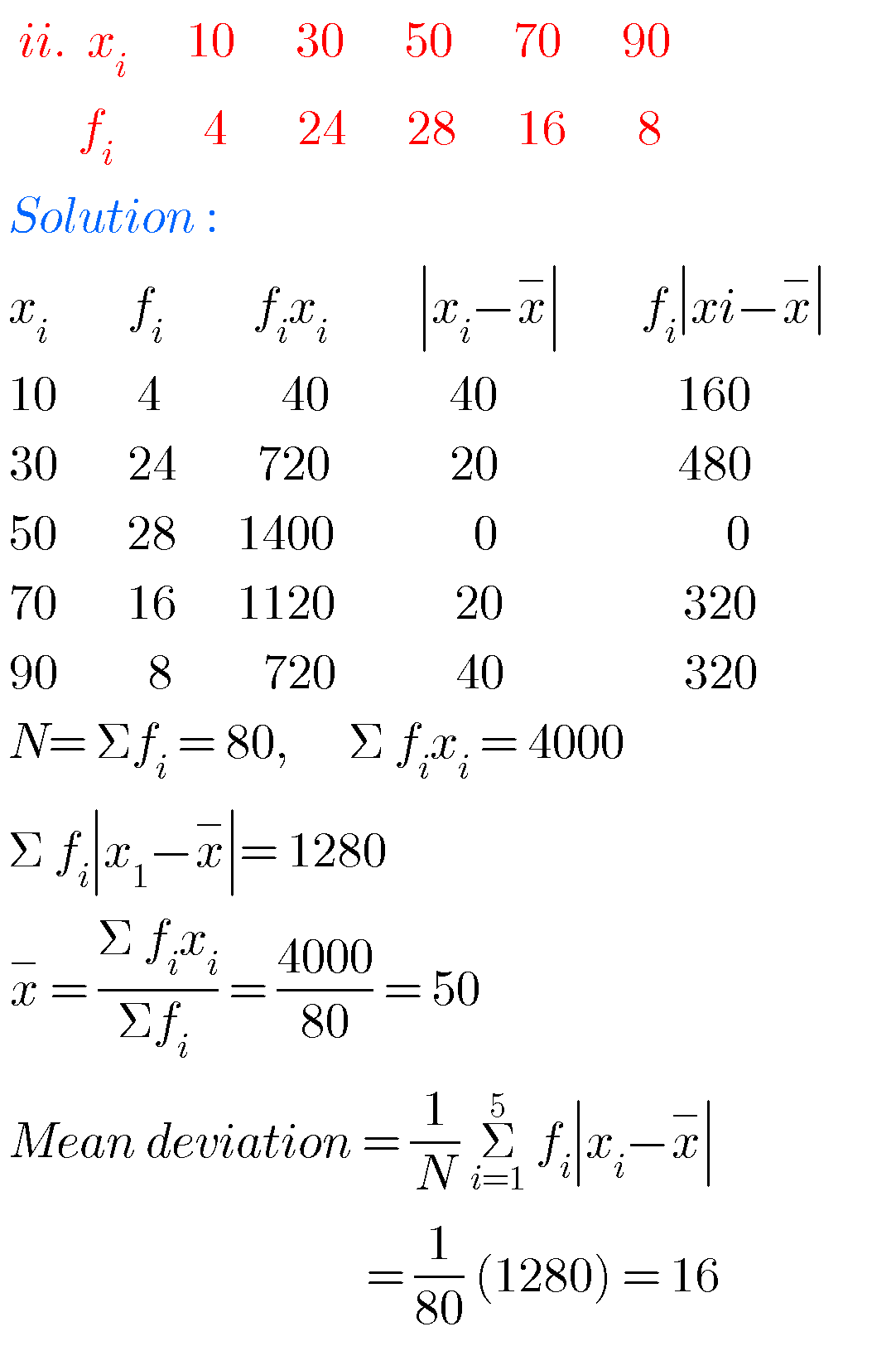## Maths solutions for inter measures of dispersion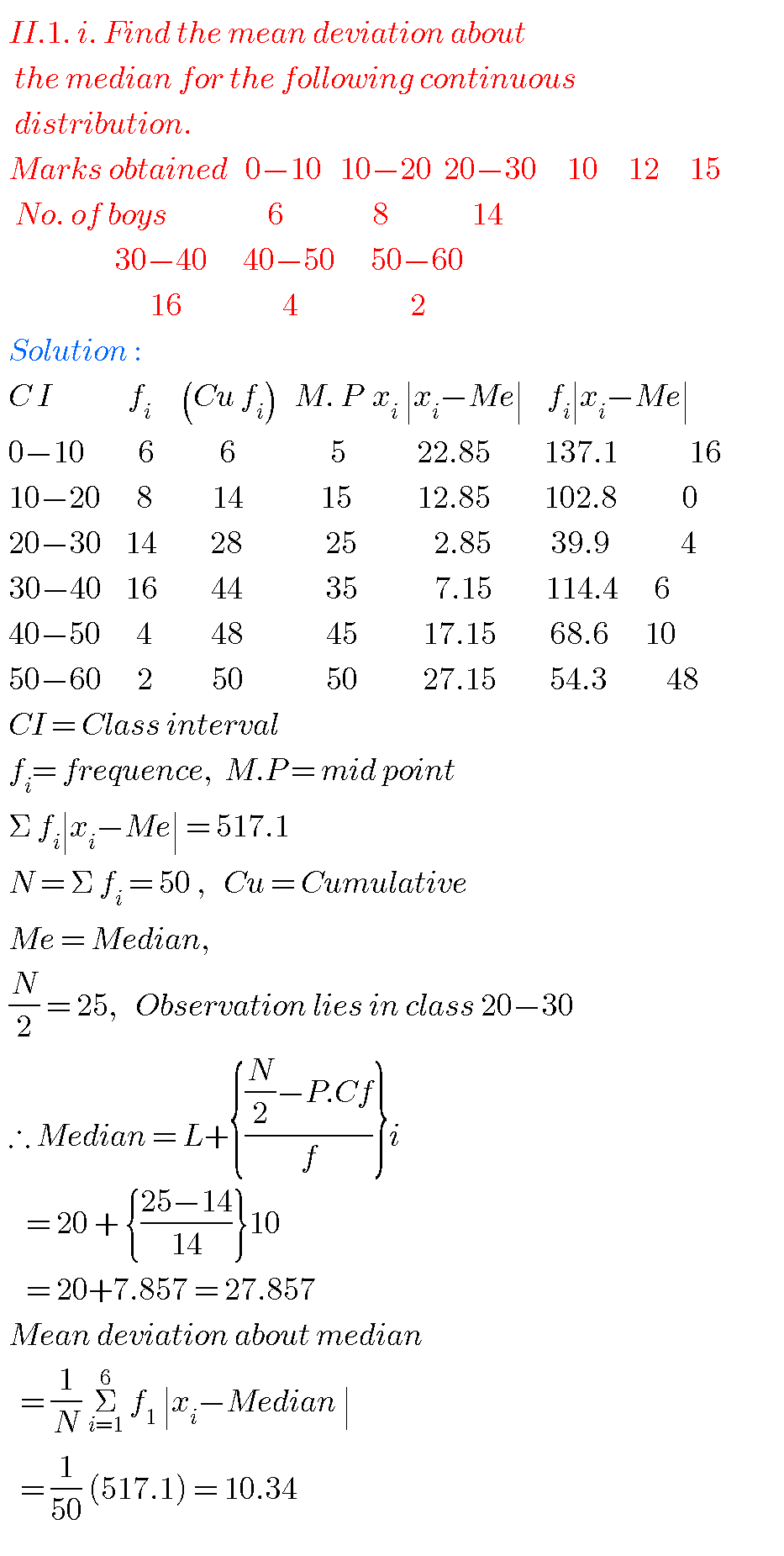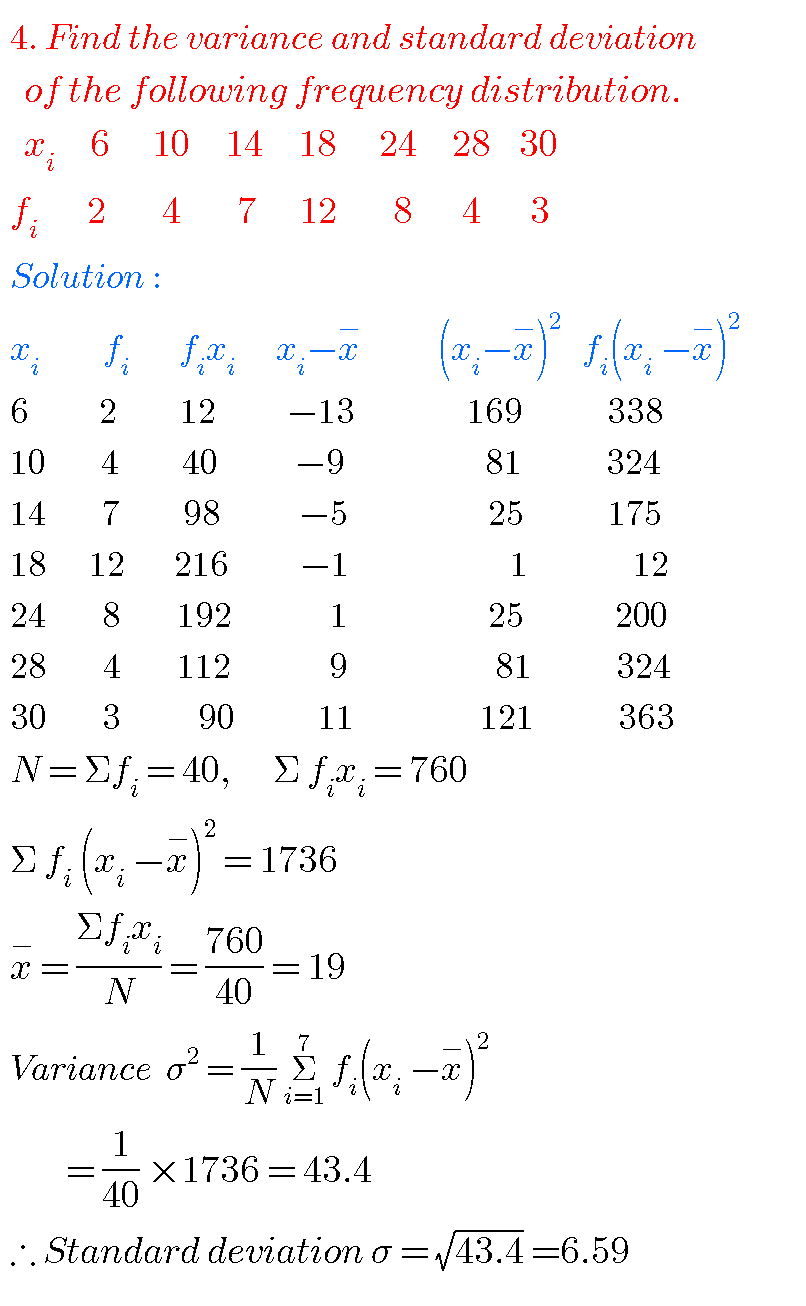### Solutions for measures of dispersion inter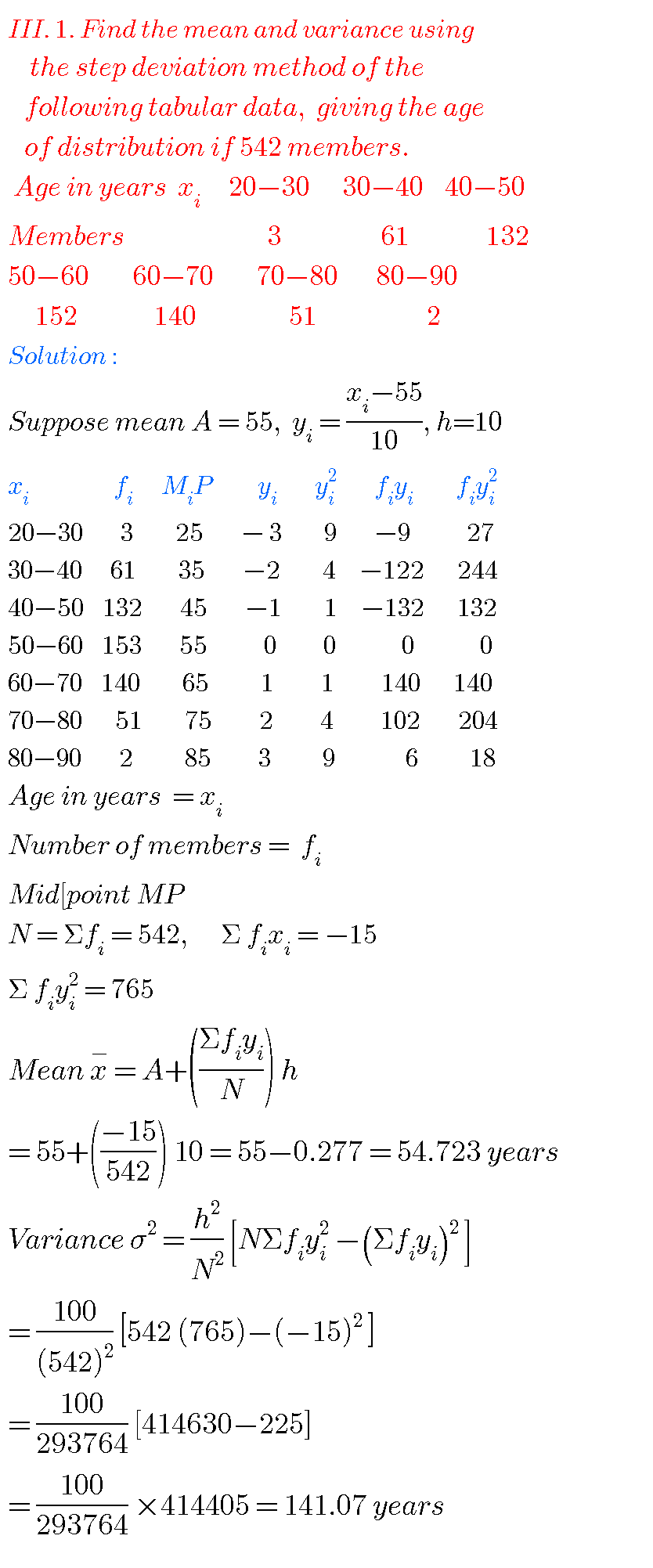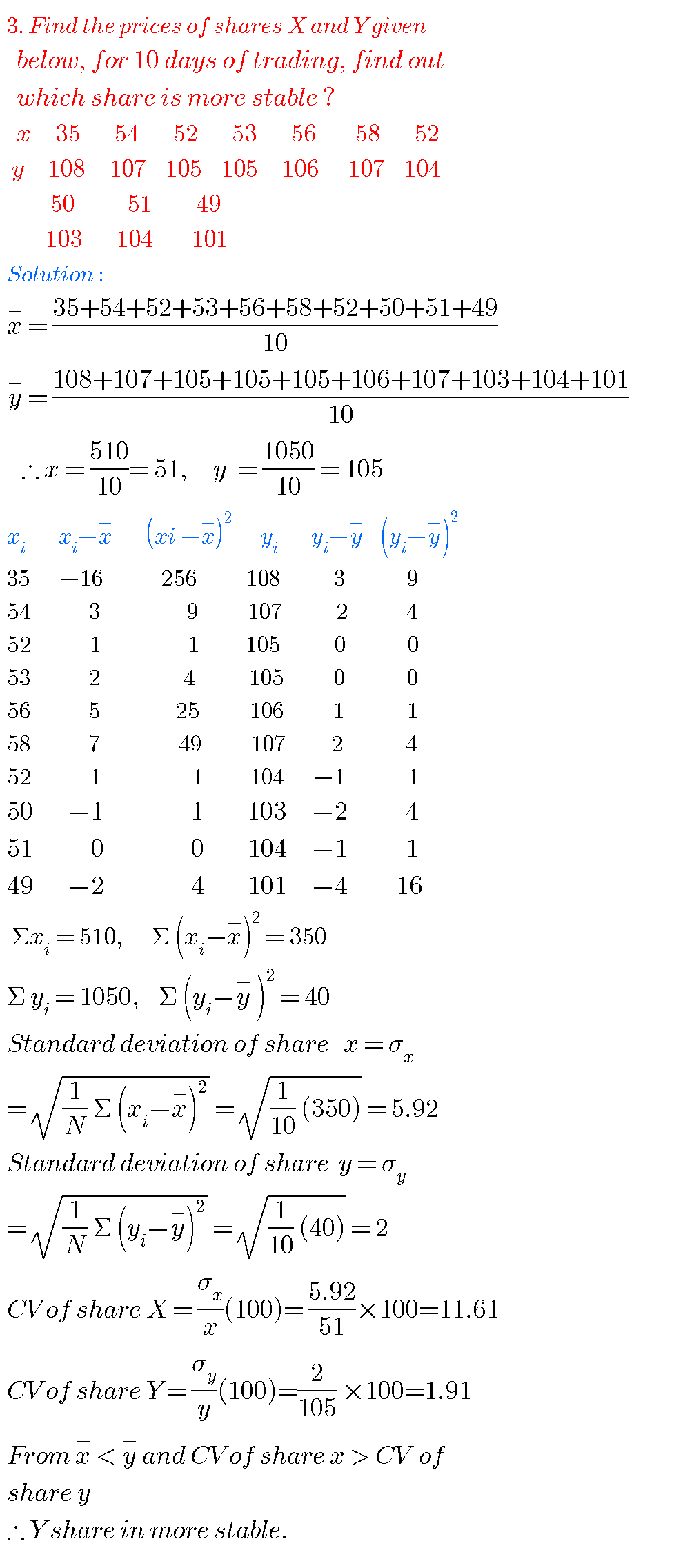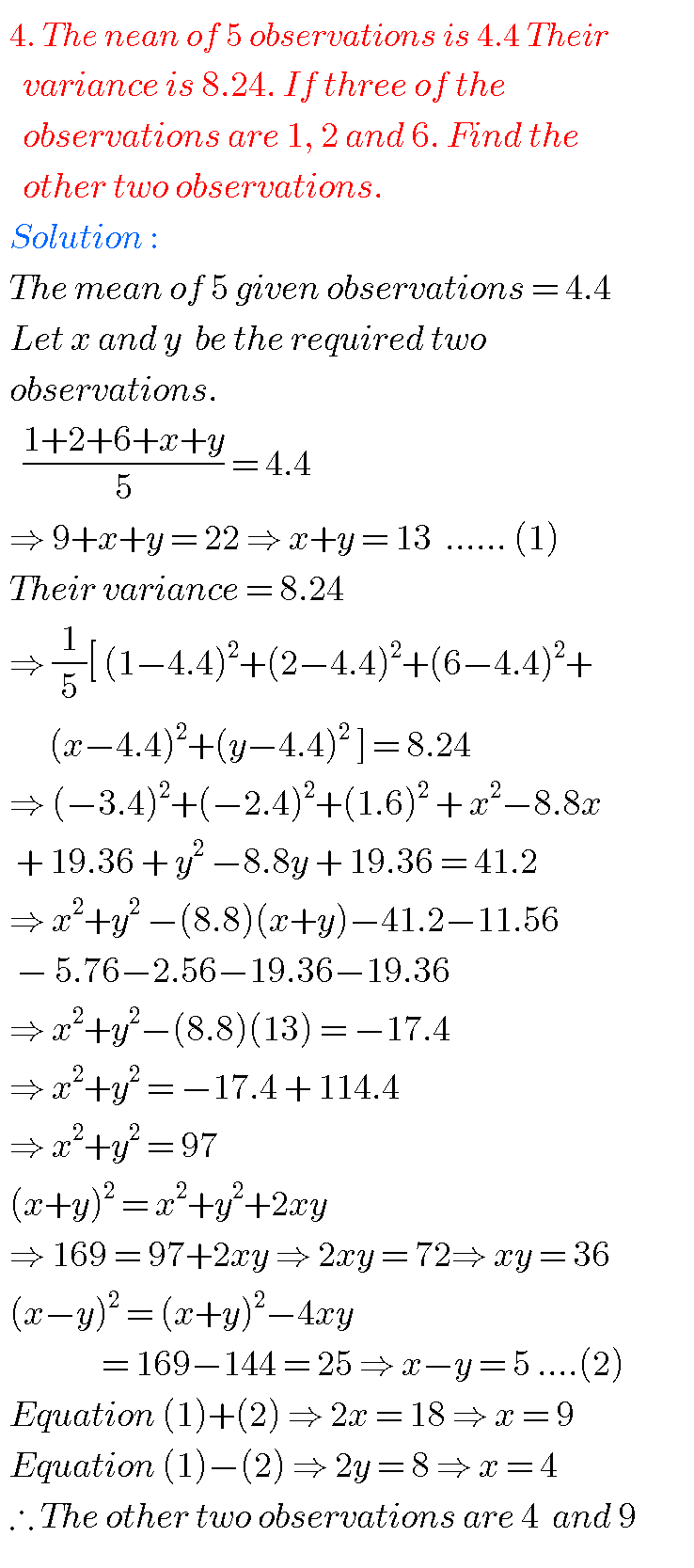Note ;  Observe the solutions and try them in your own method.

Inter maths 1b solutions

Inter 1a exercise 6(a) solutions

Inter maths 1a exercise 6(b) solutions

class 10 maths polynomials solutions

Ncert maths class 8 exponents and powers

Ncert maths class 7 fractions and decimals

Ncert maths class 6 playing with numbers

Ncert solutions for maths class 7 rational numbers

SSC class 10 solutions for maths

2. Sets

ou can see the solutions for text book Maths 1A

Functions

Exercise 1(a)

Exercise 1(b)

Exercise 1(c)

Mathematical Induction

Exercise 2(a)

Matrices

Exercise 3(a)

Exercise 3(b)

Exercise 3(c)

Exercise 3(d)

Exercise 3(e)

Exercise 3(f)

Exercise 3(g)

Exercise 3(h)

Exercise 3(i)

Exercise 4(a)

Exercise 4(b)

Product of Vectors

Exercise 5(a)

Exercise 5(b)

Exercise 5(c)

Trigonometric Ratios up to Transformations

Exercise 6(a)

Exercise 6(b)

Exercise 6(c)

Exercise 6(d)

Exercise 6(e)

Exercise 6(f)

Trigonometric Equations

Exercise 7(a)

Inverse Trigonometric Equations

Exercise 8(a)

Hyperbolic Functions

Exercise 9(a)

Properties of Triangles

Exercise 10(a)

Exercise 10(b)

Maths 1B

Transformation of axes

Exercise 2(a)

Three Dimensional Coordinates

Exercise 5(a)

Exercise 5(b)

Direction Cosines and Direction Ratios

Exercise 6(a)

Exercise 6(b)

The Plane

Exercise 7(a)

Limits and continuity

Exercise 8(a)

Exercise 8(b)

Exercise 8(d)

Exercise 8(e)